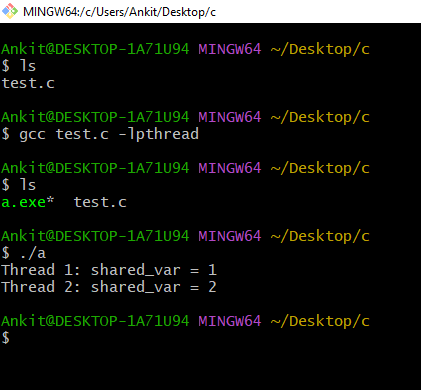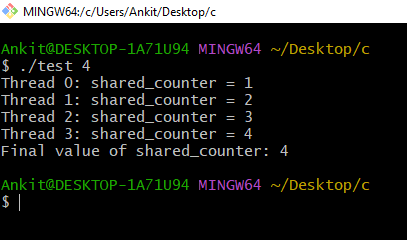Open in App
Not now

# C Program to Show Thread Interface and Memory Consistency Errors

• Difficulty Level : Medium
• Last Updated : 17 Jan, 2023

Thread interface and Memory consistency errors are demonstrated in this program. Threads are a way for a program to split itself into two or more concurrently running tasks. This means that a program can perform multiple operations at the same time, rather than having to execute them one after the other.

A thread is a separate flow of execution in a program. This means that a program with multiple threads can have multiple parts that are executed concurrently. In the context of a C program, a threaded interface is a set of functions and data structures that allow the creation and management of threads.

Example:

## C

 `// C program which  uses two threads to print "Hello" and``// "World" to the console simultaneously``#include ``#include `` ` `// Function that will be executed by the first thread``void``* hello_thread_function(``void``* arg)``{``    ``// Print "Hello" to the console``    ``printf``(``"Hello\n"``);`` ` `    ``// Return NULL to indicate successful execution of the``    ``// thread``    ``return` `NULL;``}`` ` `// Function that will be executed by the second thread``void``* world_thread_function(``void``* arg)``{``    ``// Print "World" to the console``    ``printf``(``"World\n"``);`` ` `    ``// Return NULL to indicate successful execution of the``    ``// thread``    ``return` `NULL;``}`` ` `int` `main()``{``    ``// Create the first thread``    ``pthread_t hello_thread;``    ``pthread_create(&hello_thread, NULL,``                   ``hello_thread_function, NULL);`` ` `    ``// Create the second thread``    ``pthread_t world_thread;``    ``pthread_create(&world_thread, NULL,``                   ``world_thread_function, NULL);`` ` `    ``// Wait for the first thread to finish execution``    ``pthread_join(hello_thread, NULL);`` ` `    ``// Wait for the second thread to finish execution``    ``pthread_join(world_thread, NULL);`` ` `    ``// Return success``    ``return` `0;``}`

Output:

```Hello
World```

Explanation: In this program, we have used the pthread_create() function to create two threads that execute the hello_thread_function and world_thread_function functions, respectively. These functions simply print “Hello” and “World” to the console.

Since the two threads run concurrently, the output of this program may be either “Hello\nWorld\n” or “World\nHello\n”, depending on which thread finishes first. This is because the two threads are independent and there is no guarantee about the order in which they will be executed.

One potential problem with using threads is that they can introduce memory consistency errors. This happens when multiple threads try to access and modify the same memory location simultaneously, without proper synchronization. For example, if two threads try to increment the same counter at the same time, one of the updates may be lost, resulting in an incorrect final value of the counter.

## Memory Consistency Errors

Memory consistency errors are a type of runtime error that can occur in programs with multiple threads. These errors occur when different threads have inconsistent views of the program’s memory, resulting in unexpected behavior or runtime errors.

Example:

## C

 `// C program to demonstrates the ``// use of threads and memory``// consistency errors``#include ``#include `` ` `// Global variable shared by all threads``int` `shared_var = 0;`` ` `// Function executed by the first thread``void``* thread_func1(``void``* arg)``{``    ``// Increment the shared variable by 1``    ``shared_var++;`` ` `    ``// Print the value of the shared variable``    ``printf``(``"Thread 1: shared_var = %d\n"``, shared_var);`` ` `    ``return` `NULL;``}`` ` `// Function executed by the second thread``void``* thread_func2(``void``* arg)``{``    ``// Increment the shared variable by 1``    ``shared_var++;`` ` `    ``// Print the value of the shared variable``    ``printf``(``"Thread 2: shared_var = %d\n"``, shared_var);`` ` `    ``return` `NULL;``}`` ` `int` `main(``int` `argc, ``char``* argv[])``{``    ``pthread_t thread1, thread2;`` ` `    ``// Create the first thread``    ``if` `(pthread_create(&thread1, NULL, thread_func1, NULL)``        ``!= 0) {``        ``fprintf``(stderr, ``"Error creating thread 1\n"``);``        ``return` `1;``    ``}`` ` `    ``// Create the second thread``    ``if` `(pthread_create(&thread2, NULL, thread_func2, NULL)``        ``!= 0) {``        ``fprintf``(stderr, ``"Error creating thread 2\n"``);``        ``return` `1;``    ``}`` ` `    ``// Wait for the threads to finish``    ``if` `(pthread_join(thread1, NULL) != 0) {``        ``fprintf``(stderr, ``"Error joining thread 1\n"``);``        ``return` `1;``    ``}``    ``if` `(pthread_join(thread2, NULL) != 0) {``        ``fprintf``(stderr, ``"Error joining thread 2\n"``);``        ``return` `1;``    ``}`` ` `    ``return` `0;``}`

Output:

```Thread 1: shared_var = 1Explanation: This program creates two threads, each of which increments a shared variable by 1, and then prints its value. Since the threads access and modify the shared variable simultaneously, there is a potential for memory consistency errors. Depending on the order in which the threads are executed, the final value of the shared variable may not be what we expect.

Example 2:

## C

 `// C program to create a global variable shared_counter``// that will be shared among the threads. Each thread will``// increment this variable by 1, and then print its thread``// ID and the updated value of shared_counter.``#include ``#include ``#include `` ` `// Global variable that will be shared among threads``int` `shared_counter = 0;`` ` `// Function that will be executed by each thread``void``* thread_function(``void``* thread_id)``{``    ``// Get the thread ID``    ``pthread_t tid = (pthread_t)thread_id;`` ` `    ``// Increment the shared counter``    ``shared_counter++;`` ` `    ``// Print the thread ID and the updated value of the``    ``// shared counter``    ``printf``(``"Thread %ld: shared_counter = %d\n"``, (``long``)tid,``           ``shared_counter);`` ` `    ``// Return NULL to indicate successful execution of the``    ``// thread``    ``return` `NULL;``}`` ` `int` `main(``int` `argc, ``char``* argv[])``{``    ``// Check if the number of arguments is correct``    ``if` `(argc != 2) {``        ``printf``(``"Usage: %s \n"``, argv);``        ``exit``(EXIT_FAILURE);``    ``}`` ` `    ``// Get the number of threads to create from the command``    ``// line arguments``    ``int` `num_threads = ``atoi``(argv);`` ` `    ``// Create an array of pthread_t structures to store the``    ``// thread IDs``    ``pthread_t* threads = (pthread_t*)``malloc``(``        ``num_threads * ``sizeof``(pthread_t));`` ` `    ``// Create the specified number of threads``    ``for` `(``int` `i = 0; i < num_threads; i++) {``        ``int` `status = pthread_create(&threads[i], NULL,``                                    ``thread_function,``                                    ``(``void``*)threads[i]);``        ``if` `(status != 0) {``            ``printf``(``"Error: pthread_create() returned error "``                   ``"code %d\n"``,``                   ``status);``            ``exit``(EXIT_FAILURE);``        ``}``    ``}`` ` `    ``// Wait for all threads to finish execution``    ``for` `(``int` `i = 0; i < num_threads; i++) {``        ``int` `status = pthread_join(threads[i], NULL);``        ``if` `(status != 0) {``            ``printf``(``"Error: pthread_join() returned error "``                   ``"code %d\n"``,``                   ``status);``            ``exit``(EXIT_FAILURE);``        ``}``    ``}`` ` `    ``// Free the memory allocated for the thread IDs``    ``free``(threads);`` ` `    ``// Print the final value of the shared counter``    ``printf``(``"Final value of shared_counter: %d\n"``,``           ``shared_counter);`` ` `    ``// Return success``    ``return` `0;``}`

Output:

```Thread 0: shared_counter = 1
Final value of shared_counter: 4```

Explanation: When multiple threads try to access and modify the same variable simultaneously, there is a potential for memory consistency errors to occur. For example, if two threads try to increment shared_counter at the same time, one of the updates may be lost.The output of the code will depend on the value of num_threads passed as a command line argument. The code will create num_threads threads and each thread will increment the shared variable shared_counter by 1. At the end, the final value of shared_counter will be printed.For example, if num_threads is 4, the output may look like this:

To avoid memory consistency errors, we need to use synchronization mechanisms such as mutexes or semaphores to ensure that only one thread can access and modify the shared memory at a time. There are several approaches that can be used to detect thread interface and memory consistency errors in a program. Some of these approaches include:

1. Using a thread-safe programming language or libraries: One way to avoid thread interface and memory consistency errors is to use a programming language or libraries that are specifically designed to be thread-safe. This means that the language or libraries provide built-in mechanisms to ensure that threads do not interfere with each other’s data and that memory is consistently accessed by all threads.
2. Using synchronization mechanisms: Another approach to avoid thread interface and memory consistency errors is to use synchronization mechanisms such as mutexes, semaphores, and monitors. These mechanisms allow threads to coordinate their access to shared data and ensure that only one thread is able to access the data at a given time. This can prevent race conditions and other types of thread interface and memory consistency errors.
3. Using transactions: In some cases, it may be possible to use transactions to ensure that memory accesses by multiple threads are atomic and consistent. Transactions allow multiple memory accesses to be treated as a single, indivisible operation, which can help prevent thread interface and memory consistency errors.
4. Using static analysis tools: There are also a number of static analysis tools that can be used to automatically detect thread interface and memory consistency errors in a program. These tools use a variety of techniques, such as symbolic execution and data flow analysis, to identify potential issues in a program and provide suggestions for how to fix them.

Overall, the key to avoiding thread interface and memory consistency errors is to carefully design and implement your program to ensure that threads do not interfere with each other’s data and that memory is consistently accessed by all threads. This may involve using a thread-safe programming language or libraries, synchronization mechanisms, transactions, or static analysis tools, depending on the specific needs of your program.

Example:

## C

 `// C program to use a mutex to avoid memory consistency``// errors``#include ``#include ``#include `` ` `// Global variable that will be shared among threads``int` `shared_counter = 0;`` ` `// Mutex to protect the shared counter``pthread_mutex_t shared_counter_mutex``    ``= PTHREAD_MUTEX_INITIALIZER;`` ` `// Function that will be executed by each thread``void``* thread_function(``void``* thread_id)``{``    ``// Get the thread ID``    ``long` `tid = (``long``)thread_id;`` ` `    ``// Lock the mutex to protect the shared counter``    ``pthread_mutex_lock(&shared_counter_mutex);`` ` `    ``// Increment the shared counter``    ``shared_counter++;`` ` `    ``// Print the thread ID and the updated value of the``    ``// shared counter``    ``printf``(``"Thread %ld: shared_counter = %d\n"``, tid,``           ``shared_counter);`` ` `    ``// Unlock the mutex``    ``pthread_mutex_unlock(&shared_counter_mutex);`` ` `    ``// Return NULL to indicate successful execution of the``    ``// thread``    ``return` `NULL;``}`` ` `int` `main(``int` `argc, ``char``* argv[])``{``    ``// Check if the number of arguments is correct``    ``if` `(argc != 2) {``        ``printf``(``"Usage: %s \n"``, argv);``        ``exit``(EXIT_FAILURE);``    ``}`` ` `    ``// Get the number of threads to create from the command``    ``// line arguments``    ``int` `num_threads = ``atoi``(argv);`` ` `    ``// Create an array of pthread_t structures to store the``    ``// thread IDs``    ``pthread_t* threads = (pthread_t*)``malloc``(``        ``num_threads * ``sizeof``(pthread_t));`` ` `    ``// Create the specified number of threads``    ``for` `(``int` `i = 0; i < num_threads; i++) {``        ``int` `status = pthread_create(``            ``&threads[i], NULL, thread_function, (``void``*)i);``        ``if` `(status != 0) {``            ``printf``(``"Error: pthread_create() returned error "``                   ``"code %d\n"``,``                   ``status);``            ``exit``(EXIT_FAILURE);``        ``}``    ``}`` ` `    ``// Wait for all threads to finish execution``    ``for` `(``int` `i = 0; i < num_threads; i++) {``        ``int` `status = pthread_join(threads[i], NULL);``        ``if` `(status != 0) {``            ``printf``(``"Error: pthread_join() returned error "``                   ``"code %d\n"``,``                   ``status);``            ``exit``(EXIT_FAILURE);``        ``}``    ``}`` ` `    ``// Free the memory allocated for the thread IDs``    ``free``(threads);`` ` `    ``// Print the final value of the shared counter``    ``printf``(``"Final value of shared_counter: %d\n"``,``           ``shared_counter);`` ` `    ``// Return success``    ``return` `0;``}`

Output:Explanation: In this program, we use the pthread_mutex_lock() and pthread_mutex_unlock() functions to protect the shared counter. When a thread wants to access the shared counter, it first locks the mutex, performs the necessary operations on the counter, and then unlocks the mutex. This ensures that only one thread can access the shared counter at a time, preventing memory consistency errors. In the example program, each thread first locks the mutex before incrementing the shared counter. This ensures that only one thread can increment the counter at a time and that the updates are not lost. When the thread is finished with the shared counter, it unlocks the mutex, allowing other threads to access and modify the counter. This ensures that the program maintains a consistent view of the shared memory and that all threads can work together to produce the correct final result.

My Personal Notes arrow_drop_up